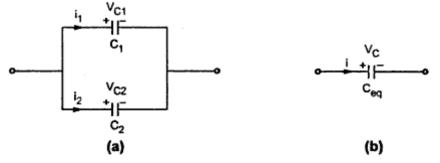### Parallel Circuits

The parallel circuit is one in which several resistance are connected across one another in such away that one terminal of each is connected to form a junction point while the remaining ends are also joined to form another junction
1. Resistors in Parallel
Consider a parallel circuit shown in the Fig. 1
In the parallel connection shown, the three resistances R1, R2 and R3 are connected in parallel and combination is connected across a source of voltage 'V'.
In parallel circuit current passing through each resistance is different. Let total current drawn is say ' I ' as shown. There are three paths for this current, one through R1 , second through R2 and third through R3 . Depending up on the values of R1, R2 and Rthe appropriate fraction of total current passes through them. These individual currents are shown as I1, I2 and I3.While the voltage across the tow ends of each resistances R1 , R2 and R3 is the same and equals the supply voltage V.Fig.  1
Now let us study current distribution. Apply Ohm's law to each resistance.
V= I1 R1   ,    V= I2 R2     , V = I3 R3
I1 = V/R1          , I2 = V/R2           I= V/R3
I = I1 + I2 +I = V/R1 + V/R2 + V/R3
= V{ 1/R1 + 1/R2 + 1/R3 )              ....................  (1)
For overall circuit if Ohm's law is applied,
V = I  Req
I = V/Req                                        ......................(2)
Where Req  = Total or equivalent resistance of the circuit.
Comparing the tow equations,
Where R is the equivalent resistance of the parallel combination.
In general if 'n' resistance are connected in parallel,
1.1 Conductance (G) :
It is known that, 1/R = G  (Conductance)  hence,
...          G = G1 +G2 +G3 +.............+ Gn                       ......For parallel circuits
Important result :
Now if n = 2, tow resistance are in parallel then,
The formula is directly used hereafter, for tow resistance in parallel.

1.2 Characteristic of Parallel Circuits
1) The same potential difference gets across all the resistance in parallel.
2) The total current gets divided into the number of paths equal to the number of resistances in parallel. The total current is always sum of all the individual currents.
I = I1 + I2 +I3 + ..........+  In
3) The reciprocal of the equivalent resistance of a parallel circuit is equal to the sum of the reciprocal of the individual resistances.
4) The equivalent resistances is the smallest of all the resistances.
R< R1     ,  R <  R ,.............., R < Rn
5) The equivalent conductance is the arithmetic addition of the individual conductances.
Key point : The equivalent resistance is smaller than the smallest of all the resistances connected in parallel.

2. Inductors in Parallel
Consider the Fig. 1(a). Tow inductors L1 and L2 are connected in parallel. The current flowing through L1 and L2 are i1 and i2 respectively. The voltage developed across L1 and L2 are VL1  and VL2  respectively. the equivalent circuits shown in Fig. 2(b).Fig.  2

For inductor we have,

For parallel combination,
V = VL1  = VL2  = and
i = i1+ i2

That means, reciprocal of equivalent inductance of the parallel combination is the sum of reciprocals of the individual inductances
For n inductances in parallel,
3. Capacitors in Parallel
Consider the Fig. 3(a). Tow capacitors C1 and C2 are connected in parallel. The current flowing through C1 and C2 are i1and i2 respectively and voltages developed across are VC1  and VC2  respectively.
The equivalent circuit is shown in the Fig. 3(b).Fig.  3

For capacitor we have,

For parallel combination,
VC1  = VC2  = V and
i = i1 + i2

Ceq  = C1 + C2
That means, equivalent capacitance of the parallel combination of the capacitance is the sum of the individual capacitance connected in series.
For n capacitor in parallel,
...                                   Ceq  = C1 + C2 + ........+ Cn